## Example Questions

### Example Question #1 : How To Multiply Square Roots

Solve for: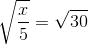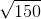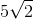Explanation:

You can "break apart" the fractional square root: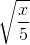into: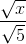Therefore, you can rewrite your equation as: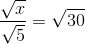Now, multiply both sides by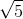: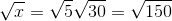Now, you can square both sides of your equation and get: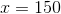### Example Question #2 : How To Multiply Square Roots

The length of a square courtyard is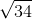feet.

What is the area of the courtyard?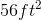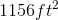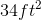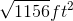Explanation:

The area of a square is length squared. In this case, that would be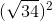which is equivalent to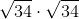. At this point, you multiply the values underneath the square root, then simplify: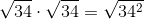.

The square root of a base squared is then just the base: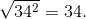Lastly, we apply the appropriate units, therefore the area of the courtyard is.

### Example Question #3 : How To Multiply Square Roots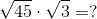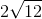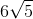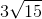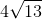Explanation:

Begin by multiplying what is under the square roots together. Remember: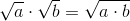Therefore: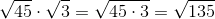We must now try to reduce the square root. Look for factors that can be taken out that can be easily square-rooted. It's a good idea to start with small square roots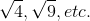. In this case, we can factor out a 9 from 135: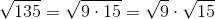Now take the square root of 9, and we are left with our answer:### Example Question #4 : How To Multiply Square Roots

Solve: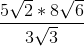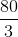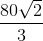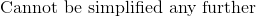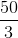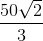Explanation:

To solve this problem, we must know how to multiply/divide with square roots. The rules involved are quite simple. When multiplying square roots together, multiply the coeffcients of the two numbers together and then multiply the two radicands together. The product of the radicand becomes the new radicand and the product of the coeffcients become the new coeffcient. The radicand is simply the number within the square root.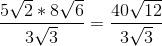Dividing square roots follows the exact same rules as multiplying square roots, divide the coeffcients together, the result is the coeffcient for final answer. Do the same for the radicands.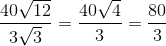Tired of practice problems?

Try live online GRE prep today.